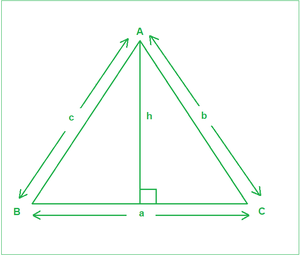Sine Rule with Derivation, Example and Implementation

• Last Updated : 13 Jul, 2021

Given angles(in degrees) A, C, and the side c, corresponding to the figure below, the task is to find the remaining two sides a and b.Examples:

Input: A = 45, C = 35, c = 23
Output:
28.35
39.49
Explanation:
a is 28.35 and b is 39.49

Input: A = 45, C = 45, c = 10
Output:
10
14.14

Approach: The idea is to use Sine rule. It states that the sides of any triangle are proportional to the sine of the angles opposite to them. a / Sin(A) = b / Sin(B) = c / Sin(C). The derivation is described below:

As is evident from the figure above:

A perpendicular of length h has been drawn on BC from A. From General trigonometric rules:

SinB=h/c——–(1)

SinC=h/b——–(2)

From the above two equations, we get:

c x SinB=b x SinC

Or b/SinB=c/SinC—–(3)

Similarly, if a perpendicular is drawn from B to AC, we can get:

a/SinA=c/SinC——-(4)

From Equations (3) and (4), we get:

a/SinA=b/SinB=c/SinC

Follow the steps below to solve the problem:

• Change the angles A and C from degrees to radians to be able to be used in the inbuilt functions.
• Calculate the angle B using the observation that sums of angles of a triangle sums up to 180 degrees.
• Use the Sine rule to calculate the sides a and b.

Below is the implementation of the above approach:

C++14

 // C++ program for the above approach#include using namespace std; // Function to calculate remaining two sidesvoid findSides(double A, double C, double c){    // Calculate angle B    double B = 180 - (A + C);     // Convert angles to their respective radians for    // using trigonometric functions    A = A * (3.14159 / 180);    C = C * (3.14159 / 180);    B = B * (3.14159 / 180);     // Sine rule    double a = (c / sin(C)) * sin(A);    double b = (c / sin(C)) * sin(B);     // Precision of 2 decimal spaces    cout << fixed << setprecision(2);     // Print the answer    cout << a << endl;    cout << b << endl;} // Driver Codeint main(){    // Input    double A = 45.0;    double C = 35.0;    double c = 23;     // Function Call    findSides(A, C, c);    return 0;}

Java

 // Java program for the above approachclass GFG{ // Function to calculate remaining two sidesstatic void findSides(double A, double C,                      double c){         // Calculate angle B    double B = 180 - (A + C);     // Convert angles to their respective    // radians for using trigonometric functions    A = A * (3.14159 / 180);    C = C * (3.14159 / 180);    B = B * (3.14159 / 180);     // Sine rule    double a = (c / Math.sin(C)) * Math.sin(A);    double b = (c / Math.sin(C)) * Math.sin(B);     // Print the answer    System.out.println(String.format("%.2f", a));    System.out.println(String.format("%.2f", b));} // Driver codepublic static void main(String[] args){         // Input    double A = 45.0;    double C = 35.0;    double c = 23;     // Function Call    findSides(A, C, c);}} // This code is contributed by abhinavjain194

Python3

 # Python3 program for the above approachimport math # Function to calculate remaining two sidesdef findSides(A, C, c):         # Calculate angle B    B = 180 - (A + C)     # Convert angles to their respective radians    # for using trigonometric functions    A = A * (3.14159 / 180)    C = C * (3.14159 / 180)    B = B * (3.14159 / 180)     # Sine rule    a = (c / math.sin(C)) * math.sin(A)    b = (c / math.sin(C)) * math.sin(B)     # Precision of 2 decimal spaces     # Print the answer    print("{0:.2f}".format(a))    print("{0:.2f}".format(b)) # Driver Code # InputA = 45.0C = 35.0c = 23 # Function CallfindSides(A, C, c) # This code is contributed by target_2

C#

 // C# program for the above approachusing System;class GFG{ // Function to calculate remaining two sidesstatic void findSides(double A, double C,                      double c){         // Calculate angle B    double B = 180 - (A + C);     // Convert angles to their respective    // radians for using trigonometric functions    A = A * (3.14159 / 180);    C = C * (3.14159 / 180);    B = B * (3.14159 / 180);     // Sine rule    double a = (c / Math.Sin(C)) * Math.Sin(A);    double b = (c / Math.Sin(C)) * Math.Sin(B);     // Print the answer    Console.WriteLine("{0:F2}",a);    Console.WriteLine("{0:F2}",b);} // Driver codepublic static void Main(String[] args){         // Input    double A = 45.0;    double C = 35.0;    double c = 23;     // Function Call    findSides(A, C, c);}} // This code is contributed by shivanisinghss2110

Javascript


Output
28.35
39.49

Time Complexity: O(1)
Auxiliary Space: O(1)

My Personal Notes arrow_drop_up